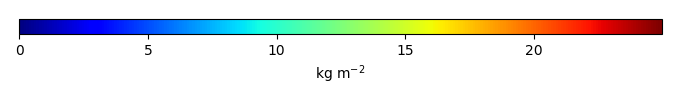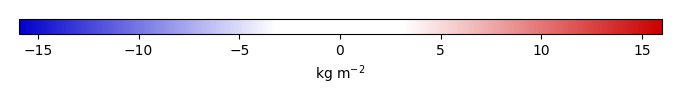# Mean State

Period Mean (original grids) [Pg]
Model Period Mean (intersection) [Pg]
Model Period Mean (complement) [Pg]
Benchmark Period Mean (intersection) [Pg]
Benchmark Period Mean (complement) [Pg]
Bias [kg m-2]
Bias Score 
Spatial Distribution Score 
Overall Score 
Benchmark [-] 30.1
CRUNCEPv7 [-] 589. 29.0 560. 29.9 0.195 0.319 0.60 0.84 0.72
GSWP3v1 [-] 487. 22.3 465. 29.9 0.195 -0.481 0.58 0.77 0.67
WATCH [-] 505. 22.6 483. 29.9 0.195 -0.504 0.61 0.74 0.67
Period Mean (original grids) [Pg]
Model Period Mean (intersection) [Pg]
Model Period Mean (complement) [Pg]
Benchmark Period Mean (intersection) [Pg]
Benchmark Period Mean (complement) [Pg]
Bias [kg m-2]
Bias Score 
Spatial Distribution Score 
Overall Score 
Benchmark [-] 1.44
CRUNCEPv7 [-] 16.4 5.84 10.4 1.41 0.0279 4.22 0.26 0.35 0.31
GSWP3v1 [-] 14.5 5.95 8.42 1.41 0.0279 4.18 0.21 0.47 0.34
WATCH [-] 11.4 4.46 6.77 1.41 0.0279 2.85 0.31 0.55 0.43
Period Mean (original grids) [Pg]
Model Period Mean (intersection) [Pg]
Model Period Mean (complement) [Pg]
Benchmark Period Mean (intersection) [Pg]
Benchmark Period Mean (complement) [Pg]
Bias [kg m-2]
Bias Score 
Spatial Distribution Score 
Overall Score 
Benchmark [-] 0.00649
CRUNCEPv7 [-] 11.9 0.0238 11.6 0.00649 0.00 0.192 0.54 0.67 0.61
GSWP3v1 [-] 10.2 0.0250 9.91 0.00649 0.00 0.233 0.58 0.75 0.67
WATCH [-] 10.8 0.0311 10.6 0.00649 0.00 0.336 0.78 0.78 0.78
Period Mean (original grids) [Pg]
Model Period Mean (intersection) [Pg]
Model Period Mean (complement) [Pg]
Benchmark Period Mean (intersection) [Pg]
Benchmark Period Mean (complement) [Pg]
Bias [kg m-2]
Bias Score 
Spatial Distribution Score 
Overall Score 
Benchmark [-] 17.4
CRUNCEPv7 [-] 17.8 17.3 0.314 17.3 0.0180 0.419 0.69 0.92 0.80
GSWP3v1 [-] 12.0 11.6 0.244 17.3 0.0180 -1.06 0.65 0.85 0.75
WATCH [-] 13.9 13.5 0.233 17.3 0.0180 -0.616 0.70 0.86 0.78
Period Mean (original grids) [Pg]
Model Period Mean (intersection) [Pg]
Model Period Mean (complement) [Pg]
Benchmark Period Mean (intersection) [Pg]
Benchmark Period Mean (complement) [Pg]
Bias [kg m-2]
Bias Score 
Spatial Distribution Score 
Overall Score 
Benchmark [-] 1.52
CRUNCEPv7 [-] 56.8 2.46 54.5 1.46 0.0621 7.01 0.56 0.88 0.72
GSWP3v1 [-] 43.6 1.80 42.0 1.46 0.0621 3.72 0.65 0.90 0.77
WATCH [-] 38.9 1.31 37.8 1.46 0.0621 1.14 0.64 0.74 0.69
Period Mean (original grids) [Pg]
Model Period Mean (intersection) [Pg]
Model Period Mean (complement) [Pg]
Benchmark Period Mean (intersection) [Pg]
Benchmark Period Mean (complement) [Pg]
Bias [kg m-2]
Bias Score 
Spatial Distribution Score 
Overall Score 
Benchmark [-] 9.78
CRUNCEPv7 [-] 3.49 3.35 0.157 9.71 0.0683 -1.61 0.49 0.57 0.53
GSWP3v1 [-] 3.04 2.93 0.149 9.71 0.0683 -1.73 0.48 0.41 0.45
WATCH [-] 3.35 3.21 0.171 9.71 0.0683 -1.67 0.48 0.43 0.45

# Temporally integrated period mean

BENCHMARK MEANMODEL MEANBIASBIAS SCORESPATIAL TAYLOR DIAGRAMMODEL COLORS# Spatially integrated regional mean

MODEL COLORS# All Models

BenchmarkCRUNCEPv7GSWP3v1WATCH